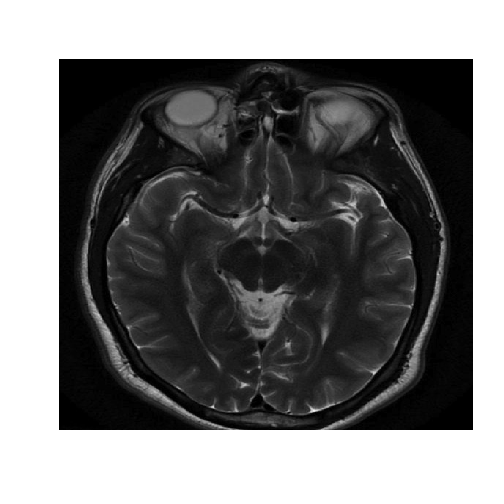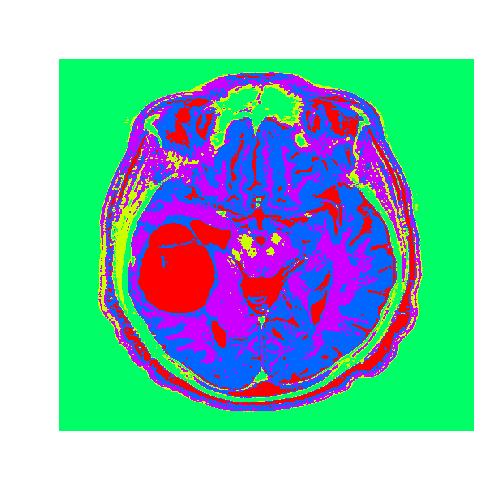# MRI image segmentation

Magnetic Resonance Imaging (MRI) is a medical image technique used to sense the irregularities in human bodies. Segmentation technique for Magnetic Resonance Imaging (MRI) of the brain is one of the method used by radiographer to detect any abnormality happened specifically for brain.

In digital image processing, segmentation refers to the process of splitting observe image data to a serial of non-overlapping important homogeneous region. Clustering algorithm is one of the process in segmentation.
Clustering in pattern recognition is the process of partitioning a set of pattern vectors in to subsets called clusters.

There are various image segmentation techniques based on clustering. One example is the K-means clustering.

### Image Segmentation

Let's try the Hierarchial clustering with an MRI image of the brain.
The healthy data set consists of a matrix of intensity values.To use hierarchical clustering we first need to convert the healthy matrix to a vector. And then we need to compute the distance matrix.

R gives us an error that seems to tell us that our vector is huge, and R cannot allocate enough memory.

Let's see the structure of the healthy vector.

The healthy vector has 365636 elements. Let's call this number n. For R to calculate the pairwise distances, it would need to calculate n*(n-1)/2 and store them in the distance matrix.
This number is 6.6844659 × 1010. So we cannot use hierarchical clustering.

Now let's try use the k-means clustering algorithm, that aims at partitioning the data into k clusters, in a way that each data point belongs to the cluster whose mean is the nearest to it.

### Analyze the clusters

To output the segmented image we first need to convert the vector healthy clusters to a matrix.
We will use the dimension function, that takes as an input the healthyClusters vector. We turn it into a matrix using the combine function, the number of rows, and the number of columns that we want.### Now we will use the healthy brain vector to analyze a brain with a tumor

The tumor.csv file corresponds to an MRI brain image of a patient with oligodendroglioma, a tumor that commonly occurs in the front lobe of the brain. Since brain biopsy is the only definite diagnosis of this tumor, MRI guidance is key in determining its location and geometry.

Now, we will apply the k-means clustering results that we found using the healthy brain image on the tumor vector. To do this we use the flexclust package.
The flexclust package contains the object class KCCA, which stands for K-Centroids Cluster Analysis. We need to convert the information from the clustering algorithm to an object of the class KCCA.The tumor is the abnormal substance here that is highlighted in red that was not present in the healthy MRI image.

### References

If you liked this post, you can share it with your followers or follow me on Twitter!